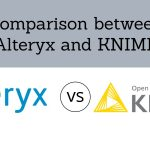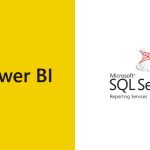[email protected] +1 203-349-9909 / +91-8080042523

### Tag

AVERAGEIF Function

### How to use Ceiling Function in Excel

The CEILING Function in Excel returns a given number rounded up to a specified multiple. CEILING Function is always used for rounding up. Its purpose is to Round a number up to nearest multiple. It returns a rounded number. What is a CEILING Function in Excel? What is the syntax for CEILING Function? Where can...

### How to use AVERAGEIFS FUNCTION in Excel

The AVERAGEIFS Function of Excel computes the average of the figures in a range that meet one or more delivered conditions. The criteria of the AVERAGEIFS Function also supports the logical operators and wildcard operators in Excel. What Is The Averageifs Function In Excel? AVERAGEIFS Function averages cells in a range that is equivalent to...

### How to use AVERAGEIF FUNCTION

The AVERAGEIF Function of Excel calculates the average of the numbers in a range that meet the supplied conditions. The measures for AVERAGEIF Function supports logical operators and wildcards for partial matching. What is an AVERAGEIF Function in Excel? What is the formula for AVERAGEIF Function? What is the example of AVERAGEIF Function? How is...